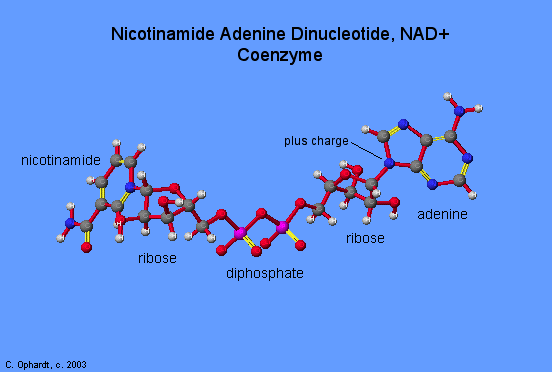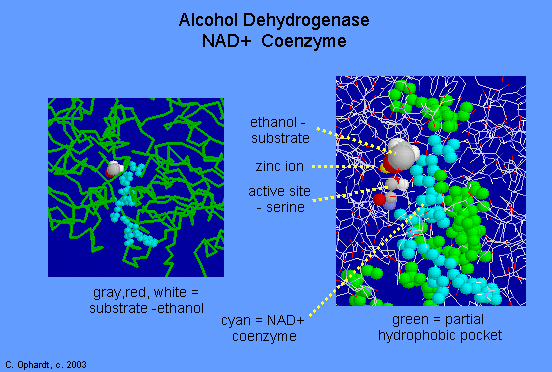Nicotinamide is from the niacin vitamin. The NAD+ coenzyme is involved with many types of oxidation reactions where alcohols are converted to ketones or aldehydes. It is also involved in the first enzyme complex 1 of the electron transport chain.The structure for the coenzyme, NAD+, Nicotinamide Adenine Dinucleotide is shown in Figure $$\PageIndex{1}$$.

One role of $$NAD^+$$ is to initiate the electron transport chain by the reaction with an organic metabolite (intermediate in metabolic reactions). This is an oxidation reaction where 2 hydrogen atoms (or 2 hydrogen ions and 2 electrons) are removed from the organic metabolite. (The organic metabolites are usually from the citric acid cycle and the oxidation of fatty acids--details in following pages.) The reaction can be represented simply where M = any metabolite.

$MH_2 + NAD^+ \rightarrow NADH + H^+ + M: + \text{energy}$

One hydrogen is removed with 2 electrons as a hydride ion ($$H^-$$) while the other is removed as the positive ion ($$H+$$). Usually the metabolite is some type of alcohol which is oxidized to a ketone.Figure $$\PageIndex{1}$$

## Alcohol Dehydrogenase

The NAD+ is represented as cyan in Figure $$\PageIndex{2}$$. The alcohol is represented by the space filling red, gray, and white atoms. The reaction is to convert the alcohol, ethanol, into ethanal, an aldehyde.

$CH_3CH_2OH + NAD^+ \rightarrow CH_3CH=O + NADH + H^+$

This is an oxidation reaction and results in the removal of two hydrogen ions and two electrons which are added to the NAD+, converting it to NADH and H+. This is the first reaction in the metabolism of alcohol. The active site of ADH has two binding regions. The coenzyme binding site, where NAD+ binds, and the substrate binding site, where the alcohol binds. Most of the binding site for the NAD+ is hydrophobic as represented in green. Three key amino acids involved in the catalytic oxidation of alcohols to aldehydes and ketones. They are ser-48, phe 140, and phe 93.Figure $$\PageIndex{2}$$: Active site of Alcohol Dehydrogenase